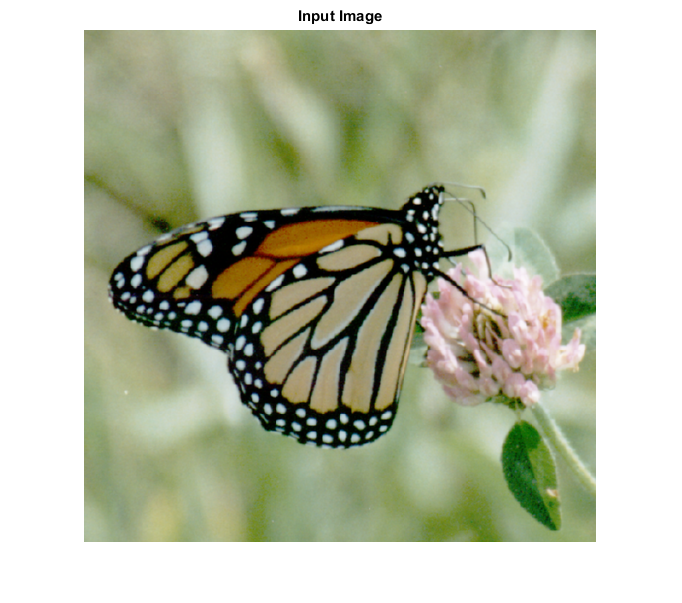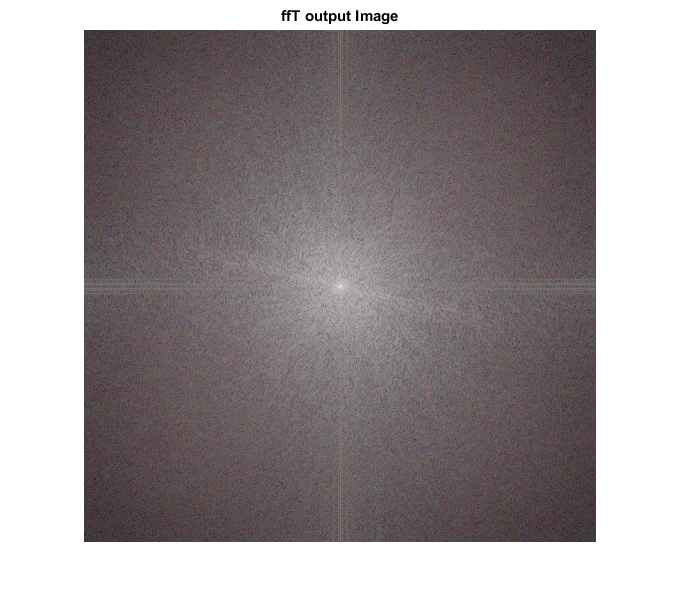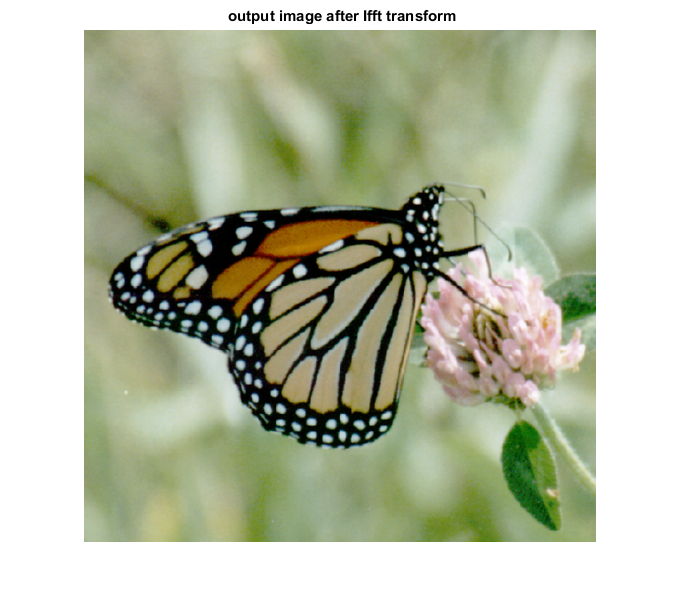# IFFT_CVIP

ifft_cvip() - perform inverse fast fourier transform.

## SYNTAX

`img = ifft_cvip(spect,block_size)`

Input parameters include:

• block_size - Should be a power of 2 or [ ].if empty,then functions finds the nearest power of 2 and zero pad the image,to that block size.Any other value smaller than image size will partiotion the image to windows of that block size and FFT will be calculated separately in each window.
• Spect - The fourier transform of the input image.

Output parameters include :

• img - Output image after inverse fft.

## DESCRIPTION

This function computes the inverse fast fourier transform of the input image. The argument block_size should match the same value given for the forward transform. For more details look at the reference below.

## REFERENCE

1.Scott E Umbaugh. DIGITAL IMAGE PROCESSING AND ANALYSIS: Applications with MATLAB and CVIPtools, 3rd Edition.

## EXAMPLE

```% Read Image

% Calling  fft function

spect = fft_cvip( I,[] );

%Calling inverse  fft function

I_hat = ifft_cvip( spect,[] );

% Display input image

figure;imshow(I);title('Input Image');

% Display output images

figure;imshow(remap_cvip(log(1+abs(spect))));title('ffT output Image');

figure; imshow(hist_stretch_cvip(I_hat,0,1,0,0));title(' output image after Ifft transform');
```